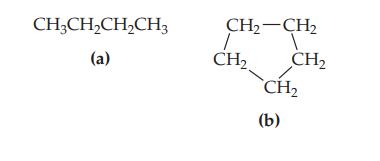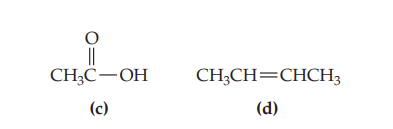×
Get Full Access to Chemistry: The Central Science - 14 Edition - Chapter 24 - Problem 24.2
Get Full Access to Chemistry: The Central Science - 14 Edition - Chapter 24 - Problem 24.2

×ISBN: 9780134414232 1274

## Solution for problem 24.2 Chapter 24

Chemistry: The Central Science | 14th Edition

• Textbook Solutions
• 2901 Step-by-step solutions solved by professors and subject experts
• Get 24/7 help from StudySoup virtual teaching assistantsChemistry: The Central Science | 14th Edition

4 5 1 276 Reviews
14
0
Problem 24.2

Which of these molecules is unsaturated? [Section 24.3]Step-by-Step Solution:
Step 1 of 3

Che 112 Week of January 28, 2018 VSEPR Shape To determine VSEPR Shape: 1) Lewis Structure 2) Determine the Central Atom, the atom least electronegative. 3) Determine the electron group arrangement (all bonds, lone pairs, and single electrons all count as single group) 4) Determine the number of lone pairs 5) Use the VSEPR Chart to find the shape (A X m n ) Polarity, Angle, and Dipole An example is C O2 . Carbon’s electronegativity is 2.5 and Oxygen’s electronegativity is 3.5. The difference in the electronegativity’s is 1.0, so the bond is polar. Anything 0.3 or greater is polar and anything less is nonpolar. There is no net dipole moment. The bond is linear and there is an 180 degree angle. A dipole moment measures the polarity of a bond. Bonds Bond Order is the number of electron pairs shared. Bond Energy is the energy needed to break attraction. Bond Length is the distance between the nuclei of bonded atoms. A higher bond order means shorter length and higher energy. A-B Bond Energy, BE, is the average enthalpy change for breaking of A-B Bond in gas phase. Bond Energy ° ∆ H = Total energy input-total energy released = Σ B E reactants Σ BE products Enthalpy, ∆ H , is almost equal to the sum of bond energies of the reactants – the sum of the bond energies of the products. Metallic Bonding The electron sea model:  Valence electrons go to delocalized “sea”  “Ions” lie in an orderly array  Held t

Step 2 of 3

Step 3 of 3

## Discover and learn what students are asking

Calculus: Early Transcendental Functions : Multiple Integration
?In Exercises 1 and 2, evaluate the integral. $$\int_{y}^{2 y}\left(x^{2}+y^{2}\right) d x$$

Calculus: Early Transcendental Functions : Differential Equations: Growth and Decay
?In Exercises 1-10, solve the differential equation. $$\frac{d y}{d x}=6-y$$

Statistics: Informed Decisions Using Data : Inference about the Difference between Two Medians: Dependent Samples
?In Problems 3–10, use the Wilcoxon matched-pairs signedranks test to test the given hypotheses at the a = 0.05 level of significance. The dependent sa

Unlock Textbook Solution